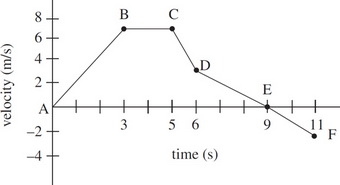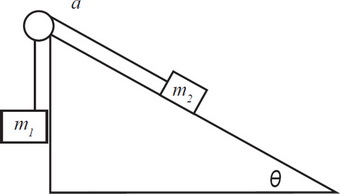# AP Physics 1 Practice Test 6

### Test Information10 questions18 minutes

1. An object is thrown horizontally to the right off a high cliff with an initial speed of 7.5 m/s. Which arrow best represents the direction of the object’s velocity after 2 seconds? (Assume air resistance is negligible.)

2.Which of the following correctly ranks the change in kinetic energy for each segment from least to greatest?

3.During which segment is the magnitude of average acceleration greatest?

4.What is the total distance traveled by the object?

5. A ball is thrown in a projectile motion trajectory with an initial velocity v at an angle θ above the ground. If the acceleration due to gravity is -g, which of the following is the correct expression of the time it takes for the ball to reach its highest point, y, from the ground?

6. A bubble in a glass of water releases from rest at the bottom of the glass and rises at acceleration a to the surface in t seconds. How much farther does the bubble travel in its last second than in its first second?

7. A person standing on a horizontal floor is acted upon by two forces: the downward pull of gravity and the upward normal force of the floor. These two forces

8. Which of the following graphs best represents the force of friction on an object starting at rest that eventually starts sliding across a level surface due to a gradually increasing horizontal force?

9. Two objects have a mass 1 kg and carry a charge of magnitude 1 C each. Which statement correctly identifies the relationship between the magnitude of the force of gravity, Fg, and the magnitude of the electric force, FE, between the objects?

10.Consider the above configuration of masses attached via a massless rope and pulley over a frictionless inclined plane. What is the acceleration of the masses?